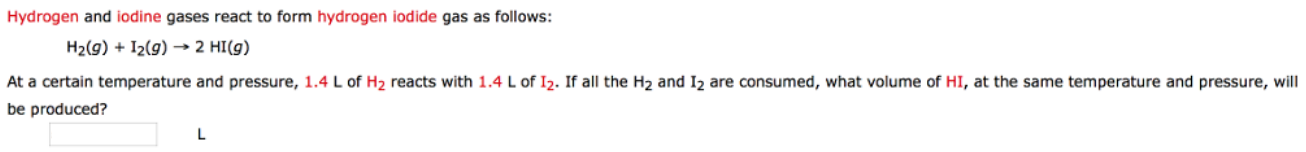Chemistry Practice Problems Gas Stoichiometry Practice Problems Solution: Hydrogen and iodine gases react to form hydrogen i...

# Solution: Hydrogen and iodine gases react to form hydrogen iodide gas as follows: H2 (g) + I2 (g) → 2HI (g) At a certain temperature and pressure 1.4 L of H 2 reacts with 1.4 L of I 2. If all the H2 and I2 are consumed, what volume of HI, at the same temperature and pressure, will be produced?

###### Problem

Hydrogen and iodine gases react to form hydrogen iodide gas as follows:

H2 (g) + I2 (g) → 2HI (g)

At a certain temperature and pressure 1.4 L of H 2 reacts with 1.4 L of I 2. If all the H2 and I2 are consumed, what volume of HI, at the same temperature and pressure, will be produced?View Complete Written Solution

Gas Stoichiometry

Gas Stoichiometry

#### Q. What is the total volume of all gases that are produced when 32.0 mL of C3H5(NO3)3 reacts as shown in the reaction below. Assume standard temp and pre...

Solved • Tue Jun 26 2018 15:14:51 GMT-0400 (EDT)

Gas Stoichiometry

#### Q. Chlorine gas is collected from the following reaction of MnO2(s) with HCl:MnO2(s) + 4HCl(aq) → MnCl2(aq) + Cl2(g) + 2H2O(l) Calculate the volume of HC...

Solved • Tue Jun 19 2018 15:19:46 GMT-0400 (EDT)

Gas Stoichiometry

#### Q. Chlorine gas reacts with fluorine gas to form chlorine trifluoride. Cl2 (g) + 3F2 (g) → 2 ClF3 (g) A 2.00 L reaction vessel, initially at 298 K, conta...

Solved • Tue Jun 19 2018 15:01:51 GMT-0400 (EDT)

Gas Stoichiometry

#### Q. Ammonium carbonate decomposes upon heating according to the following balanced equation: (NH4)2CO3 (s) → 2 NH3 (g) + CO2 (g) + H2O (g) Calculate the t...

Solved • Tue Jun 19 2018 14:58:19 GMT-0400 (EDT)